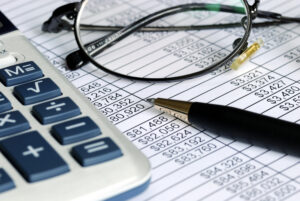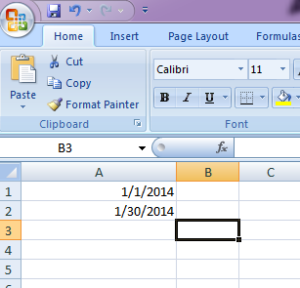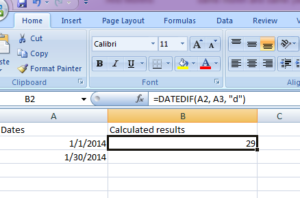Just like regular numbers, there will be times that you need to subtract dates. The problem is what unit do you want to use to subtract dates: by day, month or year? That’s where the DateDif function comes in handy. With the DateDif function, you can set a start date, a finish date and the unit you want to use for the subtraction.

The DateDif function is not the only function available in Excel. Excel uses internal functions and also lets you use VBA (Visual Basic for Applications) to automate activities in your spreadsheets. VBA is a more advanced procedure for Excel, and you’ll need to know the Visual Basic language to properly code your spreadsheet activity. However, you only need to know how to work with Excel and your rows and columns to use the DateDif function.

Learn in-depth Excel functions

The first step is to open your existing Excel spreadsheet or creating a new spreadsheet. To be the most efficient, it’s best to have a date column. You typically place your calculated results in a second column. The following image is the sample dates that will be used to perform the calculation and display the example results.In this example, the two dates used are 1/1/2014 and 1/30/2014. At a quick glance, you can calculate the amount of days, month and years (even though the result for month and years is zero).

Click the column you want to use to display the date calculation difference. In this example, column A is where the dates are located and column B will be for the date calculation.

The DateDif function uses the following syntax:

DATEDIF(start_date,end_date,unit)

The “start_date” is the first date, and the “end_date” parameter is the date you want to subtract from the “start_date” parameter. The “unit” is how you define what you want to subtract. The “unit” parameter can be “Y,” “M,” or “D” or year, month or day respectively.

New to Excel? Learn the basics at Udemy.com

You type the DateDif function calculation in the results column (in this case, it’s column B). The following image shows you an example of the DateDif function, the calculation and the results from the calculation.Notice the equal sign in front of the function. The equal sign tells Excel that you want to use a function and you are not typing the actual text. If you eliminate the equal sign, Excel assumes you are actually using the text and not trying to calculate a result.

Get started with Excel fundamental concepts

If you notice in the screenshot, the result is 29. Also notice that the DateDif function uses the column letters and row numbers. In this calculation, “A3” and “A2” are used. If you have several columns you wanted to calculate, you could highlight several rows and choose the “Fill Down” option. The result is that Excel copies your function calculation to several rows at once. Excel is also smart enough to know that you probably don’t want to calculate the same rows in all calculation results. Excel will then see the pattern and use the subsequent rows to do the calculations. The “Fill Down” option is one of the most convenient methods to use in Excel, especially when you have only one function you want to use against several rows.

Another great advantage to using column letters and row numbers is when you change the values in those fields, the DateDif function automatically updates the new results. If you change one date to 2015, the DateDif function runs the new values and returns “394” in the results column.

The DateDif function is just one function of many in the Excel library of tools. You can calculate dates, numbers, fractions and even manipulate strings with Excel functions. If you click the “Fx” button next to the Excel line editor, you can search for functions and find one that meets your needs for calculations. For more advanced features, look into Excel’s developer tools, which use VBA to program more advanced features in an Excel spreadsheet. With VBA, you can create a mini application for your workbooks that use buttons, automation and imports data from other sources such as external databases. You can even integrate Excel with an Access database. With Excel, you can do many of the functions that are offered in much more expensive applications.

Page Last Updated: March 2014

### Top courses in Excel

Microsoft Excel - Excel from Beginner to Advanced
Kyle Pew, Office Newb
4.6 (400,474)
Bestseller
Microsoft Excel: Data Analysis with Excel Pivot Tables
Maven Analytics, Chris Dutton
4.6 (55,245)
Bestseller
Microsoft Excel: Data Visualization, Excel Charts & Graphs
Maven Analytics, Chris Dutton
4.6 (42,961)
Bestseller
Microsoft Excel Pro Tips: Go from Beginner to Advanced Excel
Maven Analytics, Chris Dutton
4.6 (4,967)
Bestseller
Microsoft Excel: Beginner to Advanced for 2023
4.6 (2,219)
Microsoft Excel - Excel from Beginner to Advanced 2023
Warrick Klimaytys
4.6 (8,066)
Bestseller
Microsoft Excel Certification Exam Prep: MO-201 Excel Expert
Maven Analytics, Enrique Ruiz
4.7 (1,894)
Bestseller